# Fredholm spectrum

(diff) ← Older revision | Latest revision (diff) | Newer revision → (diff)

A bounded operatoron a complex Hilbert space is said to be essentially left invertible (respectively, essentially right invertible) if there exists a bounded operatorsuch that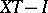(respectively,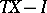) has finite rank. An operator that is essentially left or right invertible is also called a semi-Fredholm operator, andis a Fredholm operator if it is both left and right essentially invertible. F.V. Atkinson proved that an operator is Fredholm if and only if it has closed range and the spaces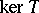and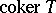have finite dimension. For a semi-Fredholm operatorone defines the index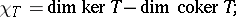this is an integer or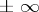. The set of semi-Fredholm operators is open, and the functionis continuous (thus locally constant). Moreover,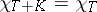ifis a compact operator;is finite ifis Fredholm. Let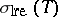be the set of complex scalarssuch that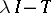is not semi-Fredholm;is a closed subset of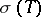(cf. Spectrum of an operator). The difference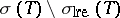is called the semi-Fredholm spectrum of, and a valuein this set belongs to the Fredholm spectrum ofifis Fredholm. Ifis one of the connected components of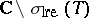, then the numberis constant for; call it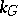. If, thenis entirely contained in the semi-Fredholm spectrum of(in the Fredholm spectrum if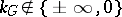). If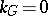, then eitheris contained in the Fredholm spectrum of, or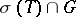has no accumulation points in. More generally, I.C. Gohberg showed thatis constant for, with the possible exception of a countable set with no accumulation points in; the dimension is greater ifis one of the exceptional points.

An interesting calculation of the Fredholm spectrum was done by Gohberg whenis a Toeplitz operator (cf. also Toeplitz matrix; Calderón–Toeplitz operator) whose symbolis a continuous function on the unit circle. In this caseis the range of, andequals minus the winding number ofaboutif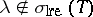.

The presence of a semi-Fredholm spectrum is related with the notion of quasi-triangularity introduced by P.R. Halmos. An operatoris quasi-triangular if it can be written aswhereis compact and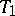is triangular in some basis. R.G. Douglas and C.M. Pearcy showed thatcannot be quasi-triangular if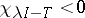for somein the semi-Fredholm spectrum of. Quite surprisingly, the converse of this statement is also true, as shown by C. Apostol, C. Foiaş and D. Voiculescu. Subsequent developments involving the Fredholm spectrum include the approximation theory of Hilbert-space operators developed by Apostol, D. Herrero, and Voiculescu.

The notion of Fredholm spectrum can be extended to operators on other topological vector spaces, to unbounded operators, and to-tuples of commuting operators. A different generalization is to define the Fredholm spectrum for elements in von Neumann algebras. The algebra of bounded operators on a Hilbert space is a factor of type. An appropriate notion of finite-rank element exists in any factor of type, and this leads to a corresponding notion of Fredholm operator and Fredholm spectrum. Analogues have been found in other Banach algebras as well.

The Fredholm property was also defined in a non-linear context by S. Smale. A differentiable mapping (cf. also Differentiation of a mapping) between two open sets in a Banach space is Fredholm if its derivative at every point is a linear Fredholm operator. This leads to the notion of a Fredholm mapping on an infinite-dimensional manifold. Smale used this notion to extend to infinite dimensions certain results of A. Sard and R. Thom.

How to Cite This Entry:
Fredholm spectrum. Encyclopedia of Mathematics. URL: http://encyclopediaofmath.org/index.php?title=Fredholm_spectrum&oldid=17870
This article was adapted from an original article by H. Bercovici (originator), which appeared in Encyclopedia of Mathematics - ISBN 1402006098. See original article